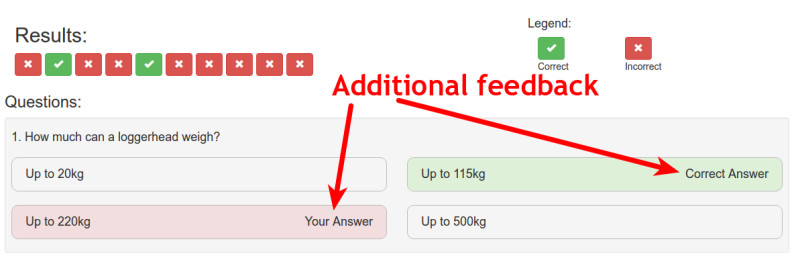# AngularJS Quiz App Tutorial Part 21 – A New Way To Use Ng-Class

## Deja Vu + A New Way To Use Ng-Class

Much like the previous part, the markup in this part will be very similar to that of the markup in the quiz controller. But we will spice things up a bit by adding new elements and showing a different way of using ng-class – using a function with ng-class instead of an object with name:value pairs.

If you want to see the app for yourself, check it out here

The git repo can be found here.

The next part can be found here

Below the row we created in the last part we will continue to create the markup for the question area of the results section.Inside the {{}} syntax of the h4 we will need to reference the active question again to ensure the correct data is showing. This means we will need to create an active question variable on the results controller.

Now that that’s done we can write the code into that h4. It will be much like the code in the h4 of the quiz controller so if you don’t quite understand what is going on here go back to the part where we created this markup in the quiz controller. Continuing on, we will also ng-repeat all the possible answers of the active question.

If you have seen the final application, you will notice that the answers in the results section display “correct answer” and if you gave a different answer it will display “your answer” on that on to let you know what answer you gave and what the actual correct answer was.To implement that we need to create the two sets of text and ng-show then depending on the conditions of each possible answer.

## Fancy Expressions and a Function With Ng-Class

The expressions in the above code snippet may look a bit daunting initially but let’s walk through them.

Starting with the “Correct Answer” text. The expression in the ng-show is comparing the \$index (which is the current index of the ng-repeat) to the correct answer for this particular question. We are doing this by referencing the correctAnswers property that we added to the quizMetrics factory earlier. Using activeQuestion to get the answer for the current active question.

The next condition to the expression is checking if this answer is in fact that answer that the user gave. We do this by referencing the selected property on the question, which of course stores the user’s answer for that particular question.

Both of these conditions have to be true, for the “Your Answer” text to display on the question.

### The Questions Need Backgrounds

We want to style the correct answer with a green background and if the user answered incorrectly, style their answer with a red background. We will do this using ng-class. But instead of using the object notation for ng-class like we have done in the past, we will give it a function that returns the class to display.

It calls the getAnswerClass function (which we will create shortly) and passes in the \$index from the ng-repeat. This function can then do some logic and figure out which class should be added and return that from the function. Whatever is returned by the function will be added as a class to the element by ng-class.

Heading into the results controller, let’s create the function. All we will need to do inside the function is figure out if the argument passed in (\$index) is the index of the correct answer. We will do this by referencing the array of correct answers we have used in the past.

If the argument is the correct answer, we return the green background class which is the bootstrap class of bg-success. If that conditional fails, there is an else if block that test if the argument passed in is the answer the user gave. If it is, then the class of bg-danger is returned, which is the red background.

We can have the else if simply check if the answer is the one the user gave and not check if it also isn’t the correct answer (like we did in the ng-show) because we already know the answer wasn’t correct as the first if statement failed.

### End Of The Road For This Part

There isn’t much left for us to do in the application. The next thing to do is to create the function that will allow the user to hop between questions using the buttons at the top.

See you in part 22 for that